# Pathfinder

Design of an indicative featured and speed controlled obstacle avoiding robot.

BeginnerFull instructions provided10 hours60,055

## Things used in this project

### Hardware componentsArduino UNO One of the primary components.
×1Ultrasonic Sensor - HC-SR04 (Generic) One of the primary components.
×1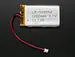Li-Ion Battery 1000mAh One of the primary components.
×2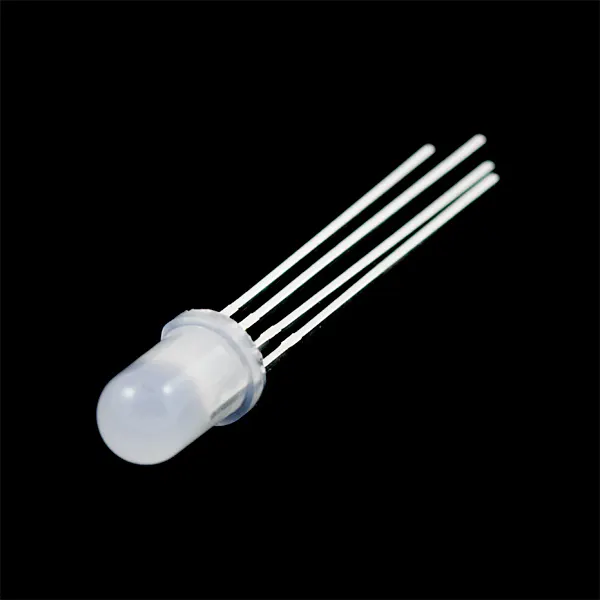RGB Diffused Common Cathode One of the primary components.
×1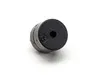Buzzer One of the necessary supplies.
×1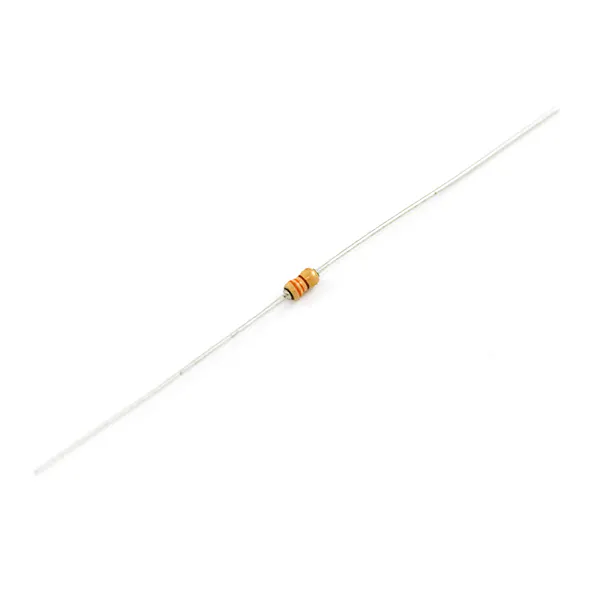Resistor 330 ohm One of the necessary supplies.
×3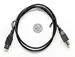USB-A to B Cable One of the necessary supplies.
×1Jumper wires (generic) One of the necessary supplies.
×20

### Software apps and online servicesArduino IDE One of the primary apps.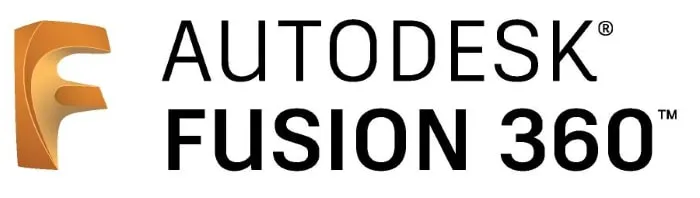Autodesk Fusion 360 One of the online services.

### Hand tools and fabrication machines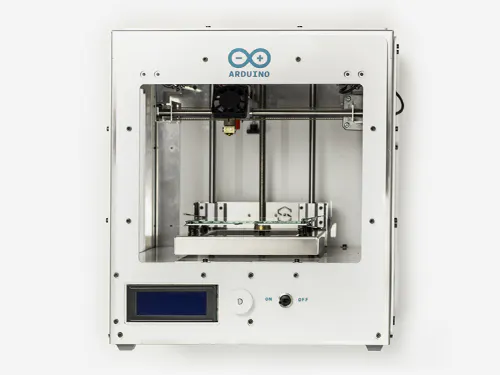Arduino Materia 101 One of the primary machines.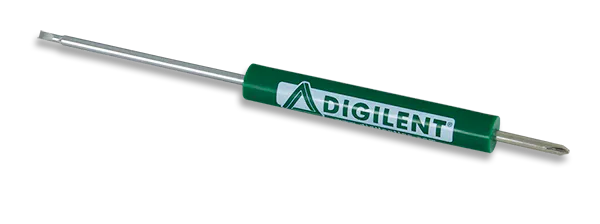Digilent Screwdriver One of the necessary tools.

## Custom parts and enclosures

### Chassis

The robot chassis, for setting up accordingly this autonomous machine.

## Schematics

### Diagram

The circuit diagram, for setting up accordingly this autonomous machine.

## Code

### Program

Arduino
The assembly program, for setting up accordingly this autonomous machine.
```#include <AFMotor.h>  // Add Adafruit Motor Shield for Arduino kit library.
#include <Servo.h>    // Add Servo Motor library.
#define BuzzPIN A0  // Assign PIN A0 as BuzzPIN (Connect Arduino UNO "A0" PIN with Buzzer "+" PIN).
#define TrigPIN A1  // Assign PIN A1 as TrigPIN (Connect Arduino UNO "A1" PIN with Ultrasonic Sonar Sensor "Trig" PIN).
#define EchoPIN A2  // Assign PIN A2 as EchoPIN (Connect Arduino UNO "A2" PIN with Ultrasonic Sonar Sensor "Trig" PIN).
#define LEDBPIN A3  // Assign PIN A3 as LEDBPIN (Connect Arduino UNO "A3" PIN with RGB Diffused Common Cathode "LEDB" PIN).
#define LEDGPIN A4  // Assign PIN A4 as LEDGPIN (Connect Arduino UNO "A4" PIN with RGB Diffused Common Cathode "LEDG" PIN).
#define LEDRPIN A5  // Assign PIN A5 as LEDRPIN (Connect Arduino UNO "A5" PIN with RGB Diffused Common Cathode "LEDR" PIN).
#define DCMROFF 25  // This sets Offset to allow differences between the two DC traction Motors.
AF_DCMotor M1 (1, MOTOR12_64KHZ); // Create DCMotor #1 using M1 output, Set to 64kHz PWM frequency.
AF_DCMotor M2 (2, MOTOR12_64KHZ); // Create DCMotor #2 using M2 output, Set to 64kHz PWM frequency.
Servo SER1; // Create Servo object to control Servo.
int Search (void) {                   // Integer type variable declaration.
float Duration = 0.0;               // Float type variable declaration.
float CM = 0.0;                     // Float type variable declaration.
digitalWrite (TrigPIN, LOW);        // TrigPIN output as 0V (Logic low level).
delayMicroseconds (2);              // Delay for 2us, Send 10 us high pulse to Ultrasonic Sonar Sensor "TrigPIN".
digitalWrite (TrigPIN, HIGH);       // TrigPIN output as 5V (Logic high level).
delayMicroseconds (10);             // Delay for 10us.
digitalWrite (TrigPIN, LOW);        // TrigPIN output as 0V (Logic low level).
Duration = pulseIn (EchoPIN, HIGH); // Start counting time, Upto again EchoPIN back to logic "High Level" and puting the "Time" into variable called "Duration".
CM = (Duration/58.8);               // Convert Distance into CM.
}
int RightDistance, LeftDistance;  // Distances on either side.
float Distance = 0.00;            // Float type variable declaration.
void setup () {               // Setup loop.
pinMode (BuzzPIN, OUTPUT);  // Declare BuzzPIN as "Output PIN".
pinMode (TrigPIN, OUTPUT);  // Declare TrigPIN as "Output PIN".
pinMode (EchoPIN, INPUT);   // Declare EchoPIN as "Output PIN".
pinMode (LEDBPIN, OUTPUT);  // Declare LEDBPIN as "Output PIN".
pinMode (LEDGPIN, OUTPUT);  // Declare LEDGPIN as "Output PIN".
pinMode (LEDRPIN, OUTPUT);  // Declare LEDRPIN as "Output PIN".
SER1.attach (10);           // Attaches the Servo on pin 10 (SER1 on the Adafruit Motor Shield for Arduino kit to the Servo object).
}
void loop () {                                    // Main loop.
SER1.write (80);                                // Tells the Servo to position at 80 degrees (Facing forward).
delay (100);                                    // Delay for 0.1s.
Distance = Search ();                           // Measuring the Distance in CM.
if (Distance < 30) {                            // If obstacle found in 30cm.
digitalWrite (BuzzPIN, HIGH);                 // BuzzPIN output as 5V (Logic high level).
digitalWrite (LEDBPIN, LOW);                  // LEDBPIN output as 0V (Logic low level).
digitalWrite (LEDGPIN, LOW);                  // LEDGPIN output as 0V (Logic low level).
digitalWrite (LEDRPIN, HIGH);                 // LEDRPIN output as 5V (Logic high level).
M1.setSpeed (100);                            // Speed down.
M2.setSpeed (100);                            // Speed down.
ChangePath ();                                // If forward is blocked Change direction.
}
else if ((Distance >= 30) && (Distance < 60)) { // If obstacle found between 30cm to 60cm.
digitalWrite (BuzzPIN, LOW);                  // BuzzPIN output as 0V (Logic low level).
digitalWrite (LEDBPIN, HIGH);                 // LEDBPIN output as 5V (Logic high level).
digitalWrite (LEDGPIN, LOW);                  // LEDGPIN output as 0V (Logic low level).
digitalWrite (LEDRPIN, LOW);                  // LEDRPIN output as 0V (Logic low level).
M1.setSpeed (150);                            // Speed increase slightly.
M2.setSpeed (150);                            // Speed increase slightly.
Forward ();                                   // Robot move to Forward direction.
}
else if ((Distance >= 60) && (Distance < 90)) { // If obstacle found between 60cm to 90cm.
digitalWrite (BuzzPIN, LOW);                  // BuzzPIN output as 0V (Logic low level).
digitalWrite (LEDBPIN, LOW);                  // LEDBPIN output as 0V (Logic low level).
digitalWrite (LEDGPIN, HIGH);                 // LEDGPIN output as 5V (Logic high level).
digitalWrite (LEDRPIN, LOW);                  // LEDRPIN output as 0V (Logic low level).
M1.setSpeed (200);                            // Speed up.
M2.setSpeed (200);                            // Speed up.
Forward ();                                   // Robot move to Forward direction.
}
else {                                          // If obstacle cannot be found in 90cm.
digitalWrite (BuzzPIN, LOW);                  // BuzzPIN output as 0V (Logic low level).
digitalWrite (LEDBPIN, HIGH);                 // LEDBPIN output as 5V (Logic high level).
digitalWrite (LEDGPIN, HIGH);                 // LEDGPIN output as 5V (Logic high level).
digitalWrite (LEDRPIN, HIGH);                 // LEDRPIN output as 5V (Logic high level).
M1.setSpeed (250);                            // Speed increase fully.
M2.setSpeed (250);                            // Speed increase fully.
Forward ();                                   // Robot move to Forward direction.
}
}
void ChangePath () {         // Path Change loop.
Stop ();                   // Robot Stop.
Backward ();               // Robot run Backward direction.
Stop ();                   // Robot Stop.
SER1.write (12);           // Check Distance to the Right.
delay (500);               // Delay for 0.5s.
RightDistance = Search (); // Set Right Distance.
delay (500);               // Delay for 0.5s.
SER1.write (160);          // Check Distance to the Left.
delay (1000);              // Delay for 1s.
LeftDistance = Search ();  // Set Left Distance.
delay (500);               // Delay for 0.5s.
delay (500);               // Delay for 0.5s.
CompareDistance ();        // Find the longest distance.
}
void CompareDistance () {                   // Distance Compare loop.
if (RightDistance > LeftDistance) {       // If Right is less obstructed.
TurnRight ();                          // Robot Turn into Right direction.
}
else if (LeftDistance > RightDistance) {  // If Left is less obstructed.
TurnLeft ();                          // Robot Turn into Left direction.
}
else {                                    // If both are equally obstructed.
TurnAround ();                        // Robot Turn Around.
}
}
void Forward () {   // Forward loop.
M1.run (FORWARD); // Turn DCMotor #1 to Forward.
M2.run (FORWARD); // Turn DCMotor #1 to Forward.
}
void Backward () {    // Backward loop.
M1.run (BACKWARD);  // Turn DCMotor #1 to Backward.
M2.run (BACKWARD);  // Turn DCMotor #2 to Backward.
delay (500);       // Delay for 1s.
}
void TurnRight () {           // Right Turn loop.
M1.run (BACKWARD);          // Turn DCMotor #1 to Backward.
M2.run (FORWARD);           // Turn DCMotor #2 to Forward.
M1.setSpeed (100+DCMROFF);  // Calibrate the Speed of DCMotor #1.
delay (300);                // Delay for 0.7s.
}
void TurnLeft () {            // Left Turn loop.
M1.run (FORWARD);           // Turn DCMotor #1 to Forward.
M2.run (BACKWARD);          // Turn DCMotor #2 to Backward.
M2.setSpeed (100+DCMROFF);  // Calibrate the Speed of DCMotor #2.
delay (300);                // Delay for 0.7s.
}
void TurnAround () {          // Trun Around loop.
M1.run (FORWARD);           // Turn DCMotor #1 to Forward.
M2.run (BACKWARD);          // Turn DCMotor #2 to Backward.
M2.setSpeed (100+DCMROFF);  // Calibrate the Speed of DCMotor #2.
delay (700);               // Delay for 2.1s.
}
void Stop () {      // Stop loop.
M1.run (RELEASE); // Release DCMotor #1.
M2.run (RELEASE); // Release DCMotor #2.
delay (100);      // Delay for 0.1s.
}
```

## Credits

### Erteza Tawsif Efaz

2 projects • 29 followers
My will is to leverage my skills and expertise in research through proper sets of game plans for the betterment of society and the economy.